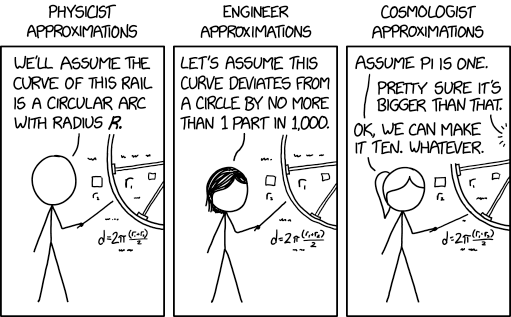# Difference between revisions of "2205: Types of Approximation"

 Types of ApproximationTitle text: It's not my fault I haven't had a chance to measure the curvature of this particular universe.

## ExplanationThis explanation may be incomplete or incorrect: Created by an APPROXIMATOR. Please mention here why this explanation isn't complete. Do NOT delete this tag too soon.If you can address this issue, please edit the page! Thanks.

In physics and engineering, problem solving typically requires approximations, as physical properties of the universe can be difficult to model. For example, in introductory physics classes, theories are introduced in frictionless environments.

In the comic, Cueball, the physicist, generally dealing with straight math, is introducing a problem with the assumption that the particular curve is a (perfectly) circular arc with a radius represented by R. Megan, the engineer, also assumes that the curve is similar to a circle, with a deviation factor of 1/1000.

The joke arises when Ponytail, the cosmologist, uses the ridiculous approximation of pi equal to 1. In actuality, pi is an irrational number, usually truncated to 3.14. Choosing the value of pi as 1, or 10, as later suggested, completely defeats the purpose of pi for describing a circle. This is a parody of the tendency of cosmology to use much rougher approximations in their work. In general, astronomers deal with masses and distances that are so vast that approximations that would be ridiculous elsewhere still yield reasonable answers in astronomy. The approximation of pi to 1 is an exaggeration of this tendency, compounded by the later approximation of both pi and 1 to 10. It may also refer to the habit astronomers have of changing the units of measure such that important constants (such as the speed of light or the gravitational constant) are equal to 1, which highly simplifies the formulas without compromising the math. In this case, the number pi is a dimensionless factor, not a directly measured quantity, which means the math will not work.

pi is defined as the ratio of the circumference of a circle divided by its diameter. This number is an irrational starting with 3.14 when the geometry is flat. But in curved spaces, the ratios are different. Almost every number can be pi depending on the curvature of the place the circle is residing. The cosmologist doesn't know the curvature of the universe, and so is approximating what pi is.

Ponytail offering to use 10 instead of 1 alludes to Randall's preferred style of solving Fermi problems, as shown in Paint the Earth. He rounds numbers to the nearest order of magnitude (1, 10, 100, etc.) using a base 10 logarithmic scale. Numbers between 0.316 and 3.16 are rounded to 1, between 3.16 and 31.6 are rounded to 10, and so on. Pi falls close to this cutoff point, and so by using this form of estimation it doesn't really matter to Ponytail whether pi is approximated to 1 or 10.

## TranscriptThis transcript is incomplete. Please help editing it! Thanks.
[Three nearly identical panels showing the lower-left portion of a wheel and hub diagram with symbols and an equation, each with a different character holding a pointer up to the diagram and a label above the panel with the character's profession:]
[Physicist Approximations]
Cueball: We'll assume the curve of this rail is a circular arc with radius R.
[Engineer Approximations]
Megan: Let's assume this curve deviates from a circle by no more than 1 part in 1,000.
[Cosmologist Approximations]
Ponytail: Assume pi is one.
Off-panel voice: Pretty sure it's bigger than that.
Ponytail: OK, we can make it ten. Whatever.add a comment! ⋅add a topic (use sparingly)! ⋅refresh comments!

# Discussion

The cosmologist is probably using Fermi's a la What-If 84: Paint the EarthOhFFS (talk) 20:34, 20 September 2019 (UTC)

In that What-If, the rounding formula for Fermi problem estimation is given as "Fermi(x) = round10(log10(x))". log10(pi) (Google search, shows calculator) is roughly .4971... so close enough that someone could do a "Fermi rounding" to either 1 or 10 and not really care one way or another. 162.158.142.118 21:19, 20 September 2019 (UTC)

As a physics Phd (though not working in astrophysics), approximating pi to 1 is not all that bad. Especially when the measurable quantities that go into the calculation usually have huge error bars.--172.68.59.120 21:03, 20 September 2019 (UTC)

Using natural units (setting c=hbar=1) is different from setting pi to 1. Using different units is always allowed and not an approximation. Setting pi to 1 on the other hand, is an approximation and is only justifiable if the other quantities in the calculation have huge uncertainty. --172.68.59.120 21:07, 20 September 2019 (UTC)

In curved spaces, size of a circle also matters a lot. Small circles will always have circumference/diameter ratio closer to pi than larger circles, no matter where they are.